Maths-
General
Easy

Question

#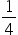litre of milk cost Rs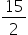. Find the cost of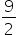litre of milk.

## The correct answer is: Rs 135

### Rs 135Cost oflitre milk= RsCost of 1 litre milk =Rs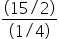=Rs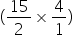= Rs 30Cost oflitre of milk =30 ×=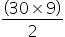= Rs 135#### With Turito Foundation.#### Get an Expert Advice From Turito.